Boyle’s Law

•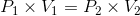• This relationship between pressure and volume in one state (P1 and V1) and pressure and volume in a second state (P2 and V2) is defined by this relationship.

• This is Boyle's Law

• This equation is used to solve Boyle's Law problems.

1. Boyle’s Law:• This equation is the one to use for solving Boyle's Law problems.

• Example #1: 2.30 L of a gas is at 725.0 mmHg pressure.

• What is its volume at standard pressure? Recall that standard pressure is 760 mmHg.

• Answer: To solve this problem we first place given values into our Boyle's law equation,  P1V1 = P2V2

•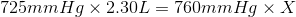• Multiply the left side and then divide by 760.0 mmHg to find x.

• The units of mmHg will cancel out.

• x is our symbol for an unknown and we need not specify units or write "x L" for "x liters". does not carry units.

• To avoid making a mistake:

• Check several times to be sure you are using the correct equation for Boyle's law and are using the right given values with units which in this case are 725 mm Hg, 2.3 L and 760 mmHg.

• Again, no need to put a unit for x, the unknown.

• So we have:

•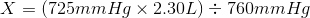2. Example #2: 4.35 L of a gas is at 1.16 atm. What pressure is obtained when the volume is 9.3 L?

• Answer: So let's use the same method as we did for problem 1.

•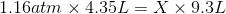•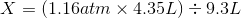3. Example #3:  Let's say this time we have 3.68 L of a gas was at an unknown pressure. However, at standard pressure, its volume was determined to be 9.20 L. We need to solve for the unknown pressure?

• Answer: This time the units of pressure was not given.  Before, in the above examples we used, mmHg (mm of Mercury) and atm (atmospheres). Since the units were not given, it's up to us and this time we'll use the kPa or "kiloPascal." value for standard pressure.

• Just as before we insert into therelationship our values to calculate the solution.

•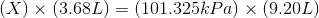•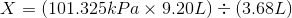Charles' Law

•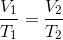• This equation  will be the basis for solving Charles' Law problems.

• Example #1: A gas is collected and found to fill 4.73 L at 35.0°C. What will be its volume at standard temperature?

• Answer: convert 35.0°C to Kelvin and you get 308 °K.

• Standard temperature is 273 °K.

• Now we insert our given values into our Charles' law equation.

•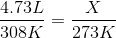• Remember to keep the correct quantities paired up: in this case 4.73 L and 308 °K and the unknown x with 273 °K

• Example #2: 8.00 L of a gas is collected at 60.0°C. What will be its volume upon cooling to 30.0°C?

• Here a wrong answer, 4.00 L. We cannot just divide by 2 because 60.0°C is twice 30 °C given that the temperature is given in Celsius; we could do it if the temperature were given in degrees Kelvin, however.  But since we're starting with Celsium we can't simply divide and be done.

• Answer:  So first we convert convert 60.0°C to 333 °K and 25.0°C to 303 °K. We MUST always convert to KELVIN!! Then plug into the equation and solve for x, like this:

•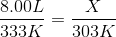•  again notice that the °K units cancel out leaving X in the proper units of volume.

• Example #3: 5.00 L of a gas is collected at 100 K and then allowed to expand to 50.0 L.

• What is the new temperature in order to maintain the same pressure?

• Here again we use Charles' Law.

•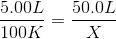Gay-Lussac's Law

•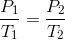• This equation is used for Gay-Lussac's Law problem.

• Again we MUST use KELVIN in these calculations, not Celsium or any other temperature unit.

• Example #1: 9.0 L of a gas is found to exert 83.0 kPa at 35.0°C. What would be the required temperature (in Celsius) to change the pressure to standard pressure?

• Answer: We must first change 35.0°C to 308.0 K and remember that standard pressure in the kiloPascal unit (kPa) is 101.325. Insert values into the equation, giving us:

•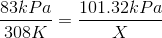• The answer is 375.9 °K, but the question asks for Celsius, so you subtract 273 to get the final answer of 102.9 °C.

• Example #2: 4.73 L of a gas is collected at 32.0°C and 625.0 mmHg. When the temperature is changed to standard conditions, what is the new pressure?

• Answer: Remember first to CONVERT TO KELVIN and  insert:

•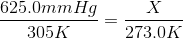Combined Gas Law

•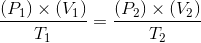• Example #1: This type of combined gas law problem is frequently encountered, especially when values are calculated at "standard temperature and pressure" or STP conditions.

• So let's say that 3.00 L of a gas is collected at 35.0°C and 705.0 mmHg. What is the volume at STP?

• Five values are provided in the problem and we need to figure our the sixth  which is our X value. As usual we need to be sure to change any temperature presented in Celsius (or any other scale) temperatures to Kelvin.

• This six variable problem can be set up with the P, V and T variables described in a table:

•  P1 = P2 = V1 = V2 = T1 = T2 =
• Then the table can be filled it in with information provided in the text of the problem. First the is the right-hand side or answer side may be filled in with the STP values:

•  P1 = P2 = 760.0 mmHg V1 = V2 = X T1 = T2 = 273 K
• On test questions, STP or standard conditions or standard temperature and pressure will be used in the problem set up.

• In the table below all the values except, of course, X are filled. in.

•  P1 = 705 mmHg P2 = 760.0 mmHg V1 =3.00 L V2 = X T1 = 308 K T2 = 273 K
• Placing these values in their correct places in the combined gas law equation: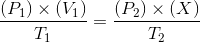allows solution for X, i.e. V2.The Ideal Gas Law

•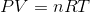• Example:

• A sample of dry gas weighing 3.1134 grams is found to occupy 3.650 L at 22.0°C and 740.0 mmHg.

• How many moles of the gas are present?

• The units for R require that the units for pressure MUST be in atm.

• Therefore, the 740.0 mm Hg must be converted first.  740.0 mm Hg ÷ 760.0 mm Hg/atm = 0.9737 atm.

• Use these values in the equation, solving for n:

• (0.9737 atm) (3.65 L) = (n) (0.08206 L atm / mol K) (295.0 K)

 atm (atmosphere) mmHg (millimeters of mercury) torr (same as mmHg) Pa (Pascal; kPa = kiloPascal) K (Kelvin) oC = degrees Celsius

 K  =  °C  +  273 1 cm3 (cubic centimeter)  =  1 mL (milliliter) 1 dm3 (cubic decimeter)  =  1 L (liter)  =  1000 mL 0.00 °C  =  273 K 1.00 atm  =  760.0 mm Hg  =  101.325 kPa  =  101,325 Pa

• Original attribution: ChemTeam and John L. Park